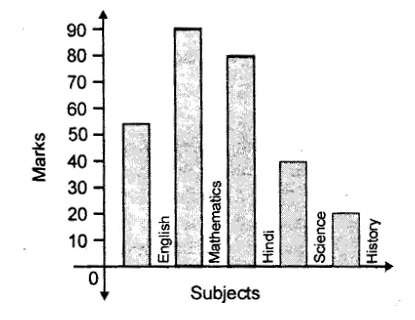# CBSE Worksheet for Chapter - 11 Statistics Class 8

## Find CBSE Worksheet for chapter-11 Statistics class 8

CLASS-8

BOARD: CBSE

Mathematic Worksheet - 11

TOPIC: Statistics

For other CBSE Worksheet for class 8 Mathematic check out main page of Physics Wallah.

OBJECTIVE:

Directions (Q.1 – Q.5): following graph shows the marks obtained by students in various subjects out of 100 marks. With the help of following graph answer the questions given below:1. At what subject is the student sharp?

(A) English

(B) Mathematics

(C) Science

(D) History

2. In which subject is the student poor?

(A) English

(B) Mathematics

(C) Science

(D) History

3. What are the average marks obtained by the student?

(A) 57

(B) 63

(C) 80

(D) 48

4. What is the percentage marks obtained by the student?

(A) 80%

(B) 63%

(C) 57%

(D) 90%

5. What is the ratio of the highest marks to the lowest marks obtained by the student?

(A) 2:11

(B) 9:2

(C) 2:9

(D) 11:2

6. The mean of 6, y, 7, x and 14 is 8, then.

(A) x + y = 13

(B) x – y = 13

(C) 2x + 3y = 13

(D) x2+y2=15(π/6)

7. If the mean of x and 1/x is M, then the mean of and 1/ is:

(A) M2

(B) M2/2

(C)  2M2 -2

(D) 2M2 -1

8. The mean of first 10 natural numbers is:

(A) 5/2

(B) 11/2

(C) 13/2

(D) 5

9. The mean of first five prime numbers is:

(A) 3

(B) 3.6

(C) 7

(D) 5.6

10. The average age of group of eight members is the same as it was 3 years ago, when a young member is substituted for an old member. The incoming member is younger to the outgoing member by:

(A) 11 years

(B) 24 years

(C) 28 years

(D) 16 years

11. Following table shows the weight of 12 students.

 Weight (in kgs) 67 70 72 73 75 Number of student 4 3 2 2 1

The mean weight is:

(A) 70.36

(B) 70.89

(C) 70.25

(D) None of these

12. Following are the marks of 30 students in a class, then the frequency of students having marks 22 is:

20, 22, 22, 25, 26, 26, 0, 10, 0, 25, 22, 23, 22, 24, 22, 24, 25, 23, 21, 25, 28, 29, 30, 27, 22, 24, 22, 25, 0, 10.

(A) 4

(B) 5

(C) 6

(D) 7

13. A cricketer has a certain average runs for 10 innings. In the 11th inning, he scores 100 runs, now his average is increased by 9 runs. Then average of 11 innings is:

10runs

(B) 20 runs

(C)11 runs

(D) 21 runs

The mean of six numbers is 15. If 2 is taken away from every number, the new mean would be:

(A)13

(B) 4

(C)17

(D) 8

1) B

2) D

3) A

4) C

5) B

6) A

7) C

8) B

9) D

10) B

11) C

12) D

13) A

14) A